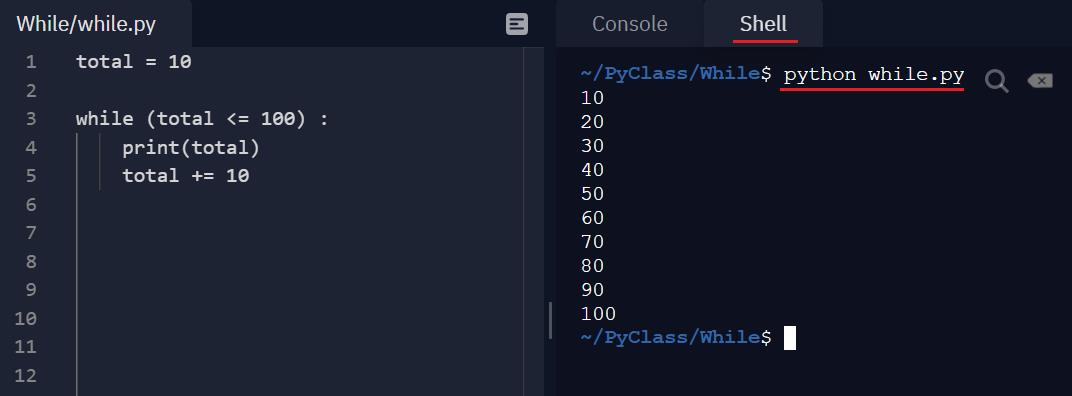### Python-23 - while 迴圈使用方法

#while(當)  條件成立(True) 的時候會一直執行

#直到條件不成立時(False)的時候跳出

 while (total <= 100) :
條件成立可執行
total = 10

while (total <= 100) :  #當
print(total)
total += 10

#第1圈條件(10 <=100)返回True,執行, 印出 total=10 並加10,  此時total為20

#第2圈條件(20 <=100)返回True,執行, 印出 total=20 並加10 , 此時total為30

#第3圈條件(30 <=100)返回True,執行, 印出 total=30 並加10 , 此時total為40

#第4圈條件(40 <=100)返回True,執行, 印出 total=40 並加10 , 此時total為50

#最後一圈加到條件 (100 <=100)返回True,執行, 印出 total=100 並加10, 此時total為110

#條件為 (110 <=100) 返回False,執行, 條件不成立 ，跳出 while迴圈YiruAtStudio - 關於我